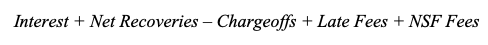Net Annualized Return (NAR) is calculated by annualizing the cumulative sum of monthly proceeds over the cumulative sum of start of month balances:where

• n is the number of months since inception
• and monthly proceeds is: